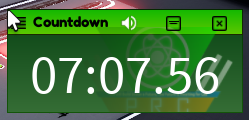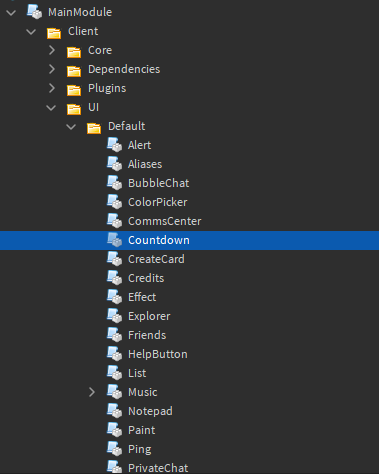# How to make a countdown with minutes and seconds in adonis

So, I saw this Adonis countdown in a game and wanted to know how to replicate it with a newer version of Adonis. Can someone look into how to do this?Something like this:

``````local textLabel = screenGui.TextLabel
local activated = true
function countdown()
local min = 0
local sec = 0
local msec = 0
while activated do
msec += 1
if msec >= 100 then
msec = 0
sec += 1
if sec >= 60 then
sec = 0
min += 1
if min >= 60 then
min = 0
end
end
end
textLabel.Text = string.format("%02i", min)..":"..string.format("%02i", sec)..":"..string.format("%02i", msec)
end
end
``````
2 Likes

Btw this is for Adonis, and not a script Gui

what is Adonis? The countdown in the image was a gui.

``````
client = nil
service = nil

return function(data)
local gTable
local tLimit = data.Time
local sImg

local isMuted = false

local tock = service.New('Sound')
tock.Volume = 0.25
tock.Looped = false
tock.SoundId = 'https://www.roblox.com/library/2734549871'

local buzzer = service.New('Sound')
buzzer.Volume = 0.25
buzzer.Looped = false
buzzer.SoundId = 'http://www.roblox.com/asset/?id=267883066'

local textSize = service.TextService:GetTextSize(tLimit, 100, Enum.Font.SourceSans, Vector2.new(math.huge,math.huge))
if textSize.X < 150 then textSize = Vector2.new(175, textSize.Y) end

local window = client.UI.Make("Window", {
Name = "Countdown";
Title = "Countdown";
Icon = client.MatIcons["Hourglass full"];
Size = {textSize.X + 30, textSize.Y + 20};
Position = UDim2.new(0, 10, 1, -(textSize.Y + 30));
OnClose = function()
tock:Stop()
buzzer:Stop()
tock:Destroy()
buzzer:Destroy()
end
})

Text = tLimit;
BackgroundTransparency = 1;
TextScaled = true;
})

Text = "";
OnClick = function()
if isMuted then
tock.Volume = 0.25
buzzer.Volume = 0.25
sImg.Image = "rbxassetid://1638551696"
isMuted = false
else
tock.Volume = 0
buzzer.Volume = 0
sImg.Image = "rbxassetid://1638584675";
isMuted = true
end
end
})

Size = UDim2.new(1,0,1,0);
Position = UDim2.new(0,0,0,0);
ScaleType = Enum.ScaleType.Fit;
Image = "rbxassetid://1638551696";
BackgroundTransparency = 1;
})

tock.Parent = label
buzzer.Parent = label
gTable = window.gTable

local startTime = os.clock()
local expectedDelay = 0
local waitTime = 1
local timeOff = 0

for i = tLimit, 1, -1 do
if gTable.Active then
tock:Play()
label.Text = i
else
break
end
wait(waitTime - timeOff)
expectedDelay += waitTime
timeOff = os.clock() - startTime - expectedDelay
end

label.Text = "0"

buzzer:Play()

for k = 0,3 do
buzzer:Play()
for i = 1, 0, -0.1 do
label.TextTransparency = i
wait(0.05)
end

for i = 0, 1, 0.1 do
label.TextTransparency = i
wait(0.05)
end
end

window:Close()
end

``````

/\ OG script

But that only displays seconds and I want in to display it as [mm:ss:ms]

Is this the whole script? What is the data variable?

It’s inside the main moduleIt looks like os gives everything between years and seconds, even tells you if its daylight savings. So you would have to get milliseconds by using os.time() + tick()%1. os.time() is only accurate to the second, so the countdown may be a little inaccurate. However, I can’t add this in the module script provided.

Can you help me figure this out? I don’t exactly understand

Does `Adonis` not have a `countdown` command? If not, I think it should be simple to implement it.

It does, but I want it to have this format, [mm:ss:ms]

I assume this to be where the time’s displayed? If so, you could simply do a bit of math to have it displayed in the format you want.

``````local mins = math.floor(i / 60)
local seconds = math.floor(i % 60)
local mili = math.floor(1e3 * (i % 1))
label.Text = string.format("%02d:%02d:%d", mins, seconds, mili)
``````